# Properties of the Riemann integral

We start by listing some properties that should seem obvious because the Riemann integral is understood as an area (mathematical).

Fact.
(i) Let f be a Riemann integrable function on [a,b]. If f ≥ 0 on [a,b], then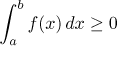.
If f is continuous on [a,b] and f > 0 on [a,b], then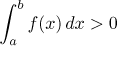.

(ii) Let f be a Riemann integrable function on [−a,a]. If f is an odd function, then.
If f is an even function, then.

(iii) Let f be a Riemann integrable function on [a,b]. If m,M are real numbers such that m ≤ f ≤ M on [a,b], then(iv) Let f and g be Riemann integrable functions on [a,b]. If f ≤ g on [a,b], then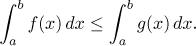(v) Let f be a Riemann integrable function on [a,b]. Then its absolute value f | is a Riemann integrable function on [a,b] andThe two properties in (ii) follow from the symmetry of graph just by looking at a picture: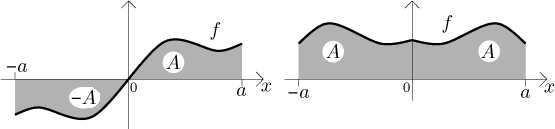Also other kinds of symmetry can be useful at times, for instance the following result should be hardly surprising:Indeed, since the graph of the sine function is symmetric,the areas above and below the x-axis cancel each other out.

The comparison properties (iii) and (iv) should be clear from this picture: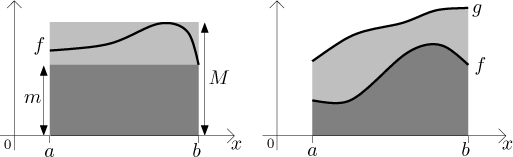While the above facts were basically just observations, the following properties are quite important:

Theorem.
(i) Let a < b<c be real numbers, let f be a function defined on the interval [a,c]. The function f is Riemann integrable on [a,c] if and only if it is Riemann integrable on both [a,b] and [b,c]; then also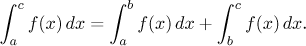(ii) Let f be a Riemann integrable function on [a,b], let k be a real number. Then the function kf is Riemann integrable on [a,b] and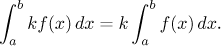(iii) Let f and g be Riemann integrable functions on [a,b]. Then the function f + g is Riemann integrable on [a,b] andThe first property should be again clear from a picture: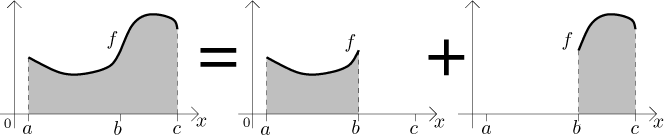The second property follows algebraically from the definition of the Riemann integral. Indeed, the constant k can be factored out of the supremum and infimum of f over individual segments, then out of the sums for upper and lower limits and finally from the infima and suprema defining the integral.

The third property is less obvious, since the supremum of a sum of two functions is definitely not equal to the sum of individual suprema. However, one has a suitable inequality there and it can be worked out. There is also a geometric argument, a curious reader may find the outline (along with some interesting tidbits concerning areas) here.